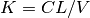• Language: en

# Analytic Compartment Functions¶

Table 70 shows the inbuilt compartment functions that are available in the DERIVATIVES section using the ‘_cl’ suffix:-

Table 70 Compartment Model Functions using ‘_cl’
Name Parameters
@iv_one_cmp_cl dose/CL/V
@dep_one_cmp_cl dose/KA/CL/V
@iv_two_cmp_cl dose/CL/V1/Q/V2
@dep_two_cmp_cl dose/KA/CL/V1/Q/V2
@iv_three_cmp_cl dose/CL/V1/Q2/V2/Q3/V3
@dep_three_cmp_cl dose/KA/CL/V1/Q2/V2/Q3/V3

The ‘_cl’ suffix means that elimination rates between compartments (K) are generally parameterised as follows:-i.e. the ratio of the clearance and volume of distribution.

## @iv_one_cmp_cl¶

Intra-venous one compartment model:-

```DERIVATIVES: |
s[CEN] = @iv_one_cmp_cl{
dose: @bolus{amt:c[AMT]},
CL: m[CL], V: m[V]}
```

This analytic model is equivalent to solving numerically:-

```DERIVATIVES: |
d[CEN] = @bolus{amt:c[AMT]} - s[CEN]*m[CL]/m[V]
```

See One Compartment Model with Intravenous Dosing for full example.

## @dep_one_cmp_cl¶

Depot and one compartment model:-

```DERIVATIVES: |
s[DEP,CEN] = @dep_one_cmp_cl{
dose: @bolus{amt:c[AMT]},
KA: m[KA], CL: m[CL], V: m[V]}
```

Numerical ordinary differential equation equivalent is:-

```DERIVATIVES: |
d[DEP] = @bolus{amt:c[AMT]} - s[DEP]*m[KA]
d[CEN] = s[DEP]*m[KA] - s[CEN]*m[CL]/m[V]
```

See One Compartment Model with Absorption for full example.

## @iv_two_cmp_cl¶

Intra-venous two compartment model:-

```DERIVATIVES: |
s[CEN,PERI] = @iv_two_cmp_cl{
dose: @bolus{amt:c[AMT]},
CL: m[CL], V1: m[V1],
Q: m[Q], V2: m[V2]}
```

Numerical ordinary differential equation equivalent is:-

```DERIVATIVES: |
d[CEN] = (
@bolus{amt:c[AMT]} - s[CEN]*m[CL]/m[V1]
- s[CEN]*m[Q]/m[V1] + s[PERI]*m[Q]/m[V2]
)
d[PERI] = s[CEN]*m[Q]/m[V1] - s[PERI]*m[Q]/m[V2]
```

See Two Compartment Model with Intravenous Dosing for full example.

## @dep_two_cmp_cl¶

Depot and two compartment model:-

```DERIVATIVES: |
s[DEP,CEN,PERI] = @dep_two_cmp_cl{
dose: @bolus{amt:c[AMT]},
KA: m[KA],
CL: m[CL], V1: m[V1],
Q: m[Q], V2: m[V2]}
```

Numerical ordinary differential equation equivalent is:-

```DERIVATIVES: |
d[DEP] = @bolus{amt:c[AMT]} - s[DEP]*m[KA]
d[CEN] = (
s[DEP]*m[KA] - s[CEN]*m[CL]/m[V1]
- s[CEN]*m[Q]/m[V1] + s[PERI]*m[Q]/m[V2]
)
d[PERI] = s[CEN]*m[Q]/m[V1] - s[PERI]*m[Q]/m[V2]
```

See Two Compartment Model with Absorption for full example.

## @iv_three_cmp_cl¶

Intra-venous three compartment model:-

```DERIVATIVES: |
s[CEN,PERI1,PERI2] = @iv_three_cmp_cl{
dose: @bolus{amt:c[AMT]},
CL: m[CL], V1: m[V1],
Q2: m[Q2], V2: m[V2],
Q3: m[Q3], V3: m[V3]
}
```

Numerical ordinary differential equation equivalent is:-

```DERIVATIVES: |
d[CEN] = (
@bolus{amt:c[AMT]}  - s[CEN]*m[CL]/m[V1]
- s[CEN]*m[Q2]/m[V1] + s[PERI1]*m[Q2]/m[V2]
- s[CEN]*m[Q3]/m[V1] + s[PERI2]*m[Q3]/m[V3]
)
d[PERI1] = s[CEN]*m[Q2]/m[V1] - s[PERI1]*m[Q2]/m[V2]
d[PERI2] = s[CEN]*m[Q3]/m[V1] - s[PERI2]*m[Q3]/m[V3]
```

See Three Compartment Model with Intravenous Dosing for full example.

## @dep_three_cmp_cl¶

Depot and three compartment model:-

```DERIVATIVES: |
s[DEP,CEN,PERI1,PERI2] = @dep_three_cmp_cl{
dose: @bolus{amt:c[AMT]},
KA: m[KA],
CL: m[CL], V1: m[V1],
Q2: m[Q2], V2: m[V2],
Q3: m[Q3], V3: m[V3]
}
```

Numerical ordinary differential equation equivalent is:-

```DERIVATIVES: |
d[DEP] = @bolus{amt:c[AMT]} - s[DEP]*m[KA]
d[CEN] = (
s[DEP]*m[KA]  - s[CEN]*m[CL]/m[V1]
- s[CEN]*m[Q2]/m[V1] + s[PERI1]*m[Q2]/m[V2]
- s[CEN]*m[Q3]/m[V1] + s[PERI2]*m[Q3]/m[V3]
)
d[PERI1] = s[CEN]*m[Q2]/m[V1] - s[PERI1]*m[Q2]/m[V2]
d[PERI2] = s[CEN]*m[Q3]/m[V1] - s[PERI2]*m[Q3]/m[V3]
```

See Three Compartment Model with Absorption for full example.

It is also possible to parametrise the rates directly using the ‘_k’ suffix, see Table 71:-

Table 71 Compartment Model Functions using ‘_k’
Name Parameters
@iv_one_cmp_k dose/KE
@dep_one_cmp_k dose/KA/KE
@iv_two_cmp_k dose/KE/K12/K21
@dep_two_cmp_k dose/KA/KE/K12/K21
@iv_three_cmp_k dose/KE/K12/K21/K13/K31
@dep_three_cmp_cl dose/KA/KE/K12/K21/K13/K31

## @iv_one_cmp_k¶

Intra-venous one compartment model:-

```DERIVATIVES: |
s[CEN] = @iv_one_cmp_k{
dose: @bolus{amt:c[AMT]},
KE: m[KE]}
```

Numerical ordinary differential equation equivalent is:-

```DERIVATIVES: |
d[CEN] = @bolus{amt:c[AMT]} - s[CEN]*m[KE]
```

## @dep_one_cmp_k¶

Depot and one compartment model:-

```DERIVATIVES: |
s[DEP,CEN] = @dep_one_cmp_k{
dose: @bolus{amt:c[AMT]},
KA: m[KA], KE: m[KE]}
```

Numerical ordinary differential equation equivalent is:-

```DERIVATIVES: |
d[DEP] = @bolus{amt:c[AMT]} - s[DEP]*m[KA]
d[CEN] = s[DEP]*m[KA] - s[CEN]*m[KE]
```

## @iv_two_cmp_k¶

Intra-venous two compartment model:-

```DERIVATIVES: |
s[CEN,PERI] = @iv_two_cmp_k{
dose: @bolus{amt:c[AMT]},
KE: m[KE], K12: m[K12], K21: m[K21]}
```

Numerical ordinary differential equation equivalent is:-

```DERIVATIVES: |
d[CEN] = (
@bolus{amt:c[AMT]} - s[CEN]*m[KE]
- s[CEN]*m[K12] + s[PERI]*m[K21]
)
d[PERI] = s[CEN]*m[K12] - s[PERI]*m[K21]
```

## @dep_two_cmp_k¶

Depot and two compartment model:-

```DERIVATIVES: |
s[DEP,CEN,PERI] = @dep_two_cmp_k{
dose: @bolus{amt:c[AMT]},
KA: m[KA], KE: m[KE],
K12: m[K12], K21: m[K21]}
```

Numerical ordinary differential equation equivalent is:-

```DERIVATIVES: |
d[DEP] = @bolus{amt:c[AMT]} - s[DEP]*m[KA]
d[CEN] = (
s[DEP]*m[KA] - s[CEN]*m[KE]
- s[CEN]*m[K12] + s[PERI]*m[K21]
)
d[PERI] = s[CEN]*m[K12] - s[PERI]*m[K21]
```

## @iv_three_cmp_k¶

Intra-venous three compartment model:-

```DERIVATIVES: |
s[CEN,PERI1,PERI2] = @iv_three_cmp_k{
dose: @bolus{amt:c[AMT]},
KE: m[KE],
K12: m[K12], K21: m[K21],
K13: m[K13], K31: m[K31]}
```

Numerical ordinary differential equation equivalent is:-

```DERIVATIVES: |
d[CEN] = (
@bolus{amt:c[AMT]} - s[CEN]*m[KE]
- s[CEN]*m[K12] + s[PERI1]*m[K21]
- s[CEN]*m[K13] + s[PERI2]*m[K31]
)
d[PERI1] = s[CEN]*m[K12] - s[PERI1]*m[K21]
d[PERI2] = s[CEN]*m[K13] - s[PERI2]*m[K31]
```

## @dep_three_cmp_k¶

Depot and three compartment model:-

```DERIVATIVES: |
s[DEP,CEN,PERI1,PERI2] = @dep_three_cmp_k{
dose: @bolus{amt:c[AMT]},
KA: m[KA], KE: m[KE],
K12: m[K12], K21: m[K21],
K13: m[K13], K31: m[K31]}
```

Numerical ordinary differential equation equivalent is:-

```DERIVATIVES: |
d[DEP] = @bolus{amt:c[AMT]} - s[DEP]*m[KA]
d[CEN] = (
s[DEP]*m[KA] - s[CEN]*m[KE]
- s[CEN]*m[K12] + s[PERI1]*m[K21]
- s[CEN]*m[K13] + s[PERI2]*m[K31]
)
d[PERI1] = s[CEN]*m[K12] - s[PERI1]*m[K21]
d[PERI2] = s[CEN]*m[K13] - s[PERI2]*m[K31]
```